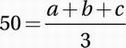# SAT Math Multiple Choice Question 662: Answer and Explanation

### Test Information

Question: 662

2. The average (arithmetic mean) of three numbers is 50. If two of the numbers have a sum of 85, what is the third number?

• A. 75
• B. 70
• C. 65
• D. 55

Explanation:

C

Data Analysis (central tendency) EASY

The average of three numbers is 50:Multiply by 3:

150 = a + b + c

Two of the numbers have a sum of 85:

85 = a + b

Substitute into the previous equation:

150 = 85 + c

Subtract 85 to find c:

65 = c